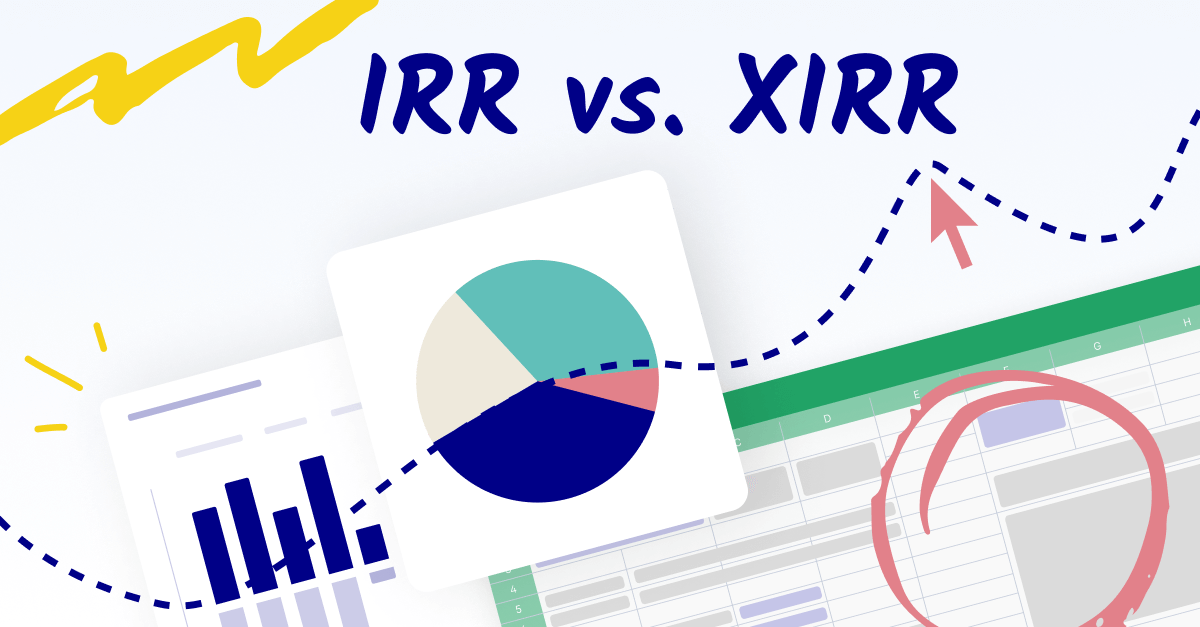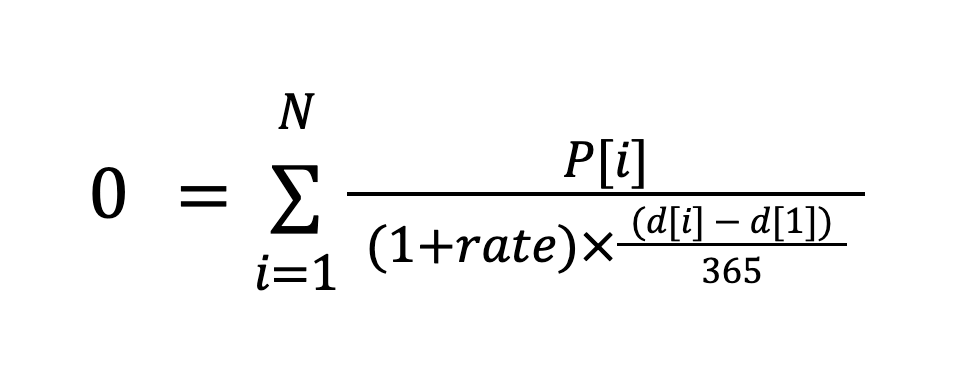Updated: November 5, 2023 |

# IRR vs. XIRR: What's the difference? When do I use them?

By

Billy RussellBilly is an expert in the FP&A space. Before joining Cube at the seed stage, Billy found success as a tax advisor at companies like Grant Thornton LLP and Gemini.com. He holds a BA and MA in Accounting from William & Mary and splits his time between NYC and New England.IRR (Internal Rate of Return) and XIRR (Extended Internal Rate of Return) are both financial metrics used to measure the profitability of an investment.

But what's the difference between them?

How do you know when to use each?

And what exactly does "the profitability of an investment" mean, anyway?#### Billy Russell

FP&A Strategist, Cube Software

###### See Cube in action

Get out of the data entry weeds and into the strategy.

## What is IRR?

IRR, or internal rate of return, measures the average return on an investment.

It's simple to understand: the higher the IRR, the better the return (and the better the investment).

The IRR is the discount rate that makes an investment's net present value (NPV) equal to zero in a discounted cash flow analysis.

It's used to measure the profitability of a single investment and is used to compare different investments.

The best use case for IRR is comparing the profitability of investments with regular cash flows. It's especially useful for comparing investments with different holding periods or cash flow patterns.

### What is net present value?

Net present value (NPV) is the difference between the present values of cash inflows and outflows over a period of time.

To oversimplify, if you expect cash inflows of \$3 and cash outflows of \$2 over a given period, your net present value for that period is \$3 - \$2 = \$1.

FP&A teams use net present value (NPV) to calculate the current value of future payments from a company, project, or investment.

You need to estimate the timing and amount of future cash flows and pick a discount rate equal to the minimum acceptable rate of return.

Here's the formula:

NPV = Cash flow ÷ [(1 + required return or discount rate)^(number of time periods)] - initial investment

In plain English, NPV is "today’s value of the expected cash flows" minus "today’s value of invested cash."

How does it relate to IRR?

Because IRR is the discount rate in the denominator that makes NPV equal to zero in a discounted cash flow analysis.

...so let's answer what a discounted cash flow analysis is.

### What is a discounted cash flow analysis?

Discounted cash flow (DCF) analysis is a valuation method to estimate the value of an investment using its expected future cash flows.

In other words, discounted cash flow uses future cash flows equal to some estimated value.

How does DCF relate to IRR?

Because IRR is part of the denominator of the estimated cash flows.

But enough of that. Let's talk about how to calculate IRR.

### How to calculate IRR

The formula for IRR looks complex, but it's pretty simple.

You calculate IRR by using the NPV formula and setting it equal to 0.

Here's the IRR formula:

​0 = NPV = [Cash inflow ÷ (1 + IRR)] - Total investment costs.

You can sum the number in brackets over many periods to get the average IRR over multiple periods.

If you're using Excel, there's an easier way to calculate IRR.

### How to calculate IRR (in Microsoft Excel)

Microsoft Excel has a built-in IRR function you can use, and it's super easy.

Here's the syntax:

IRR(values, [guess])

Where values is an array or reference to the cells you want to use to calculate the internal rate of return, and where guess is an optional argument that tells Excel where to begin its search for the optimal IRR.

If the IRR formula returns a #NUM! error, it means you should update (or remove) your guess.

And that's your IRR calculation in Excel.

### Limitations of IRR

Unfortunately, IRR isn't perfect.

IRR assumes periodic cash flows occur at regular intervals, which can lead to inaccuracies.

Likewise, since estimates in IRR and NPV can (obviously) differ from the actual results, it's best to combine IRR analysis with scenario analysis to show different possible NPVs based on varying assumptions.

This is because scenario analysis helps reduce some of the uncertainty your estimations introduce.

### Is IRR the same as ROI (return on investment?)

No, IRR and ROI are different things.

Although IRR is often referred to as the ROI of a project, it should tell you why ROI isn't the same as IRR: people use it differently.

Put another way, IRR's mathematical definition is precise and ROI's is not.## What is XIRR?

XIRR, or Extended Internal Rate of Return, is similar to IRR but is used to measure the profitability of investments with irregular cash flows.

This means it's a more accurate measure of profitability when the cash flows are not evenly spaced over time.

So XIRR is best for investments with irregular cash flows or varying holding periods.

### What are irregular future cash flows?

Irregular cash flows are unexpected.

This means they're not in any predictions---they're a surprise.

An example of an irregular cash flow might be an outlier-sized sale or deal.

### The XIRR formula

Calculating XIRR is pretty simple.

...Well, if you're using Excel. More on that in a second.

The calculations by hand can get a little hairy. Just look at the formula to calculate XIRR:Gross, right?

That's why it's better to use Excel for your XIRR calculation.

### The Excel XIRR function

Fortunately, Excel makes this super easy.

Here's the syntax:

XIRR(values, dates, [guess])

where the values argument is a series of cash flows that correspond to a schedule of payments in dates (entered with the DATE function), and guess is an optional argument of a number you think will be close to the XIRR.

### Limitations of XIRR

XIRR assumes that the cash flows are reinvested at the XIRR rate, which is unlikely to be the case in the real world. Real life is inherently more chaotic and messy.

Likewise, XIRR doesn't consider the magnitude of cash flows---so outliers in our outliers, so to speak, can throw off your XIRR model.

## What's the difference between IRR vs. XIRR?

IRR (Internal Rate of Return) and XIRR (Extended Internal Rate of Return) are both financial metrics used to measure the profitability of an investment.

IRR measures the average annual rate of return for an investment, assuming cash flows occur at regular intervals (e.g. annually or monthly). The IRR is the discount rate that makes an investment's net present value (NPV) equal to zero.

IRR measures the profitability of a single investment and is used to compare different investments.

XIRR, on the other hand, is similar to IRR but is used to measure the profitability of investments with irregular cash flows. It's a more accurate measure of profitability when the cash flows are not evenly spaced over time. It allows you to enter different cash flow dates and interpolate the internal rate of return.

IRR assumes regular cash flows and compares investments with the same holding period.

In contrast, XIRR assumes irregular cash flows and measures the profitability of investments with varying cash flow patterns and holding periods.

Both are commonly used in financial analysis and investment decision-making to evaluate the profitability and performance of an investment.

### When to use IRR vs. XIRR

Use IRR when your cash flows are regular.

• You're evaluating bonds, annuities, or other investments with consistent, periodic payments.
• The project or investment has conventional cash flows at set intervals.

Use XIRR when your cash flows are irregular.

• The investment includes irregular cash flows, such as real estate investments with variable rental incomes.
• You need to consider specific transaction dates to get an accurate return rate, as with a portfolio of stocks bought and sold at different times.

Since they're both so easy to calculate in Excel, we recommend running the numbers for each so you're better able to forecast.

## Common Pitfalls and Errors to Avoid When Using IRR and XIRR

IRR and XIRR are  metrics that are instrumental for investors and analysts in measuring the profitability of investments. However, while powerful, both IRR and XIRR can lead to misinterpretation and errors if not applied correctly. Let's explore some common pitfalls you should avoid to ensure the accuracy and reliability of your financial evaluations.

Misapplying the Metrics to Non-Standard Projects: One of the most common mistakes is using IRR or XIRR for projects with non-conventional cash flows, such as those with alternating periods of cash inflows and outflows (also known as non-normal cash flows). This can lead to multiple IRRs, making it difficult to discern the actual performance of an investment.

Overlooking Reinvestment Rate Assumptions: Both IRR and XIRR calculations are based on the assumption that intermediate cash flows are reinvested at the project's internal rate of return. This assumption is often unrealistic, as the reinvestment rate may be lower than the project's IRR. This discrepancy can lead to an overestimation of the project's profitability.

Ignoring Economic and Financial Context: Failing to consider the economic context and financial market conditions can render IRR and XIRR calculations less meaningful. For example, changes in interest rates, inflation, and market volatility can impact the project's cash flows and the applicable reinvestment rates, thus affecting the overall attractiveness of the investment.

Using IRR and XIRR in Isolation: Another mistake is relying solely on IRR or XIRR for making investment decisions. These measures should not be used in isolation as they do not account for the scale of the investment, risk, or capital cost. It's important to complement them with other metrics such as NPV, payback period, and profitability index for a comprehensive investment analysis.## Real-world applications of IRR and XIRR

Understanding the practical utility of financial metrics can transform how investments and business decisions are viewed. Let’s take a look at a few real-life uses of utilizing IRR and XIRR.

Capital Budgeting: Corporations use IRR to evaluate the profitability of capital projects, like the purchase of new machinery or expansion into new markets. IRR helps in comparing the profitability of different projects and deciding where to allocate capital.

Real Estate Development: In real estate, developers use XIRR to evaluate the potential profitability of a development project. Because cash flows in real estate are often not periodic, XIRR provides a more accurate measure of return by accounting for the specific timing of each cash flow.

Retirement Portfolios: Financial planners use XIRR to calculate the actual return on an individual's retirement portfolio. Since contributions (such as monthly 401(k) contributions and withdrawals don't happen annually but at different points throughout the year, XIRR is used to determine the annualized return on the portfolio over time, helping in assessing the efficiency of retirement savings.

## Conclusion: IRR and XIRR

Both IRR and XIRR are powerful functions in Excel that aid in investment decision-making. The choice between IRR and XIRR depends on the timing of your cash flows.

Regular intervals call for IRR, while XIRR is necessary for more complex, irregular timing. By understanding the strengths and applications of each, you can employ these functions to conduct more accurate and effective financial analyses.

You should use them with other tools, such as net present value (NPV) and return on investment (ROI), to get a complete picture of an investment's profitability.